# 预测从瞎猜开始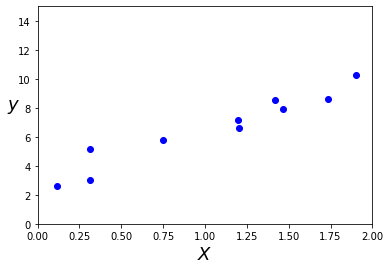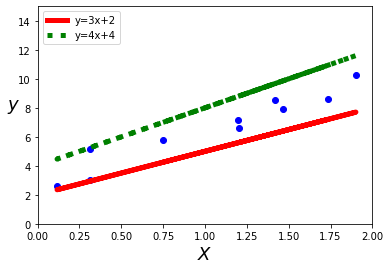# 损失函数（成本函数）

$SE = \sum{(y_{pred} -y_{true})^2}$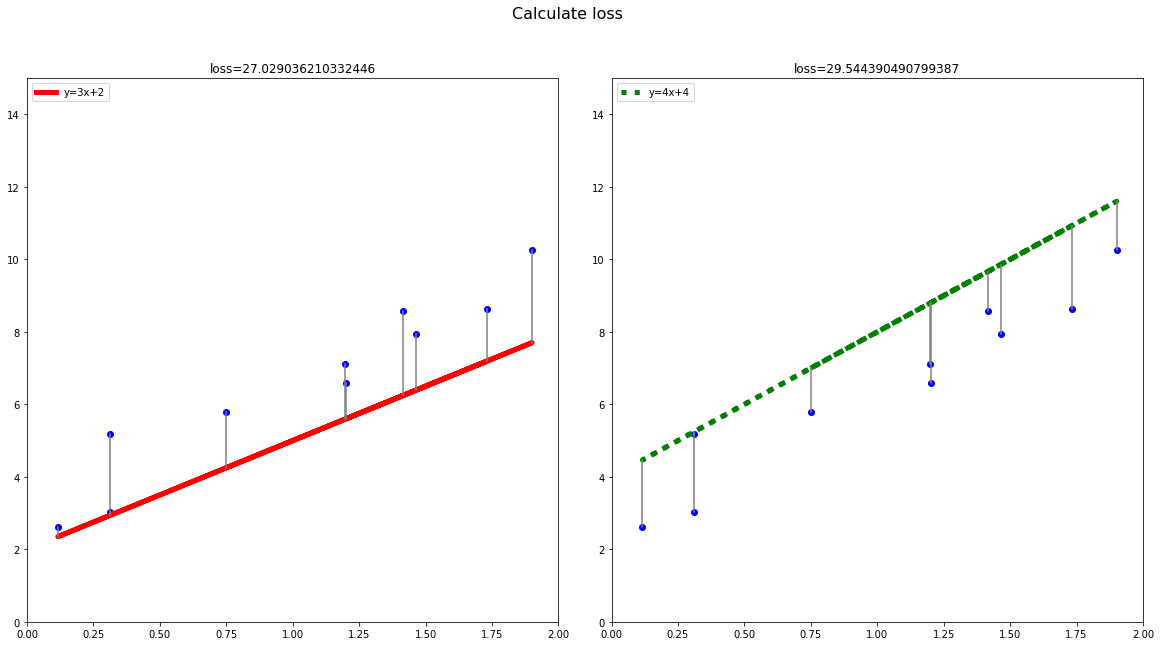# 梯度下降

$y = wx + b$# 学习率（步长）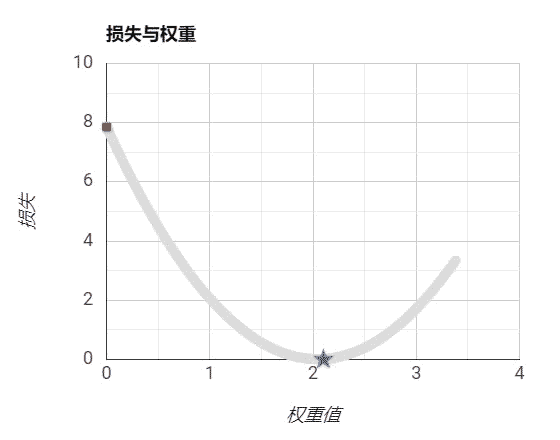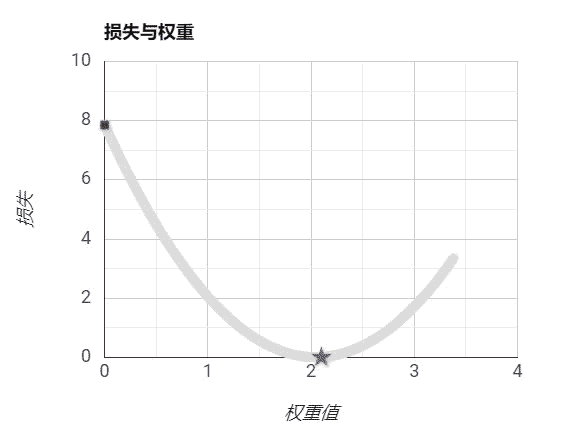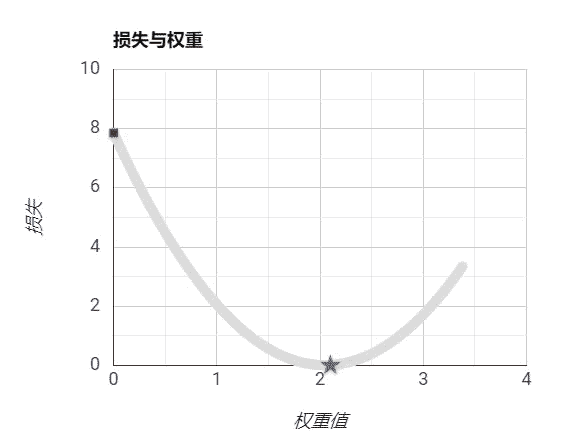# 线性回归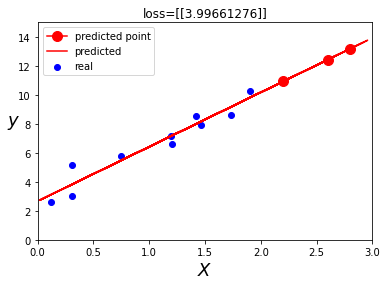# 代码实践

import numpy as np
import matplotlib.pyplot as plt

np.random.seed(42)
X = 2 * np.random.rand(10)
y = 4 + 3 * X + np.random.randn(10)

plt.plot(X, y, "bo")
plt.xlabel("$X$", fontsize=18)
plt.ylabel("$y$", rotation=0, fontsize=18)
plt.axis([0, 2, 0, 15])
plt.show()


plt.plot(X, y, "bo")
plt.plot(X, 3*X+2, "r-", lw="5", label = "y=3x+2")
plt.plot(X, 4*X+4, "g:", lw="5", label = "y=4x+4")
plt.xlabel("$X$", fontsize=18)
plt.ylabel("$y$", rotation=0, fontsize=18)
plt.axis([0, 2, 0, 15])
plt.legend(loc="upper left")
plt.show()


fig, ax_list = plt.subplots(nrows=1, ncols=2,figsize=(20,10))
ax_list.plot(X, y, "bo")
ax_list.plot(X, 3*X+2, "r-", lw="5", label = "y=3x+2")
loss = 0
for i in range(10):
ax_list.plot([X[i],X[i]], [y[i],3*X[i]+2], color='grey')
loss= loss + np.square(3*X[i]+2-y[i])
pass
ax_list.axis([0, 2, 0, 15])
ax_list.legend(loc="upper left")
ax_list.title.set_text('loss=%s'%loss)

ax_list.plot(X, y, "bo")
ax_list.plot(X, 4*X+4, "g:", lw="5", label = "y=4x+4")
loss = 0
for i in range(10):
ax_list.plot([X[i],X[i]], [y[i],4*X[i]+4], color='grey')
loss= loss + np.square(4*X[i]+4-y[i])
pass
ax_list.axis([0, 2, 0, 15])
ax_list.legend(loc="upper left")
ax_list.title.set_text('loss=%s'%loss)
fig.suptitle("Calculate loss",fontsize=16)


from sklearn.linear_model import LinearRegression
lr = LinearRegression()
lr.fit(X.reshape(-1,1),y.reshape(-1,1))

X_test = [[2.2],[2.6],[2.8]]
y_test = lr.predict(X_test)
X_pred = 3 * np.random.rand(100, 1)
y_pred = lr.predict(X_pred)
plt.scatter(X,y, c='b', label='real')
plt.plot(X_test,y_test, 'r', label='predicted point' ,marker=".", ms=20)
plt.plot(X_pred,y_pred, 'r-', label='predicted')
plt.xlabel("$X$", fontsize=18)
plt.ylabel("$y$", rotation=0, fontsize=18)
plt.axis([0, 3, 0, 15])
plt.legend(loc="upper left")
loss = 0
for i in range(10):
loss = loss + np.square(y[i]-lr.predict([[X[i]]]))
plt.title("loss=%s"%loss)
plt.show()